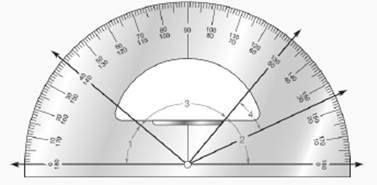Chapter P.CR, Problem 31CRElementary Geometry For College St...

7th Edition
Alexander + 2 others
ISBN: 9781337614085

Solutions

Chapter
SectionElementary Geometry For College St...

7th Edition
Alexander + 2 others
ISBN: 9781337614085
Textbook Problem

For Review Exercises 31 and 32, use the drawing below. Find the measure of each indicated angle. a) ∠ 1 b) ∠ 3To determine

a)

To find:

The measure of indicated angle 1.

Explanation

Approach:

Measuring angles:

The instrument used to measure angles is called a protractor.

For instance, the measure of RST is expressed as mRST=60° which is read as “The measure of RST is 60 degrees”

To find the degree measure of an angle using protractor:

1. Place the notch of the protractor at the point where the sides of angle meet (the vertex of angle).

2. Place the protractor along a side of the angle so that the scale reads “0

3. Using the same (outer) scale, read the angle size by reading the degree measure that corresponds to the second side of the angle.

When “0” does not correspond to one of the sides of angle, then the measure of angle is the positive difference between two numbers (on the sides of angle) on the same (outer or inner) scale

To determine

b)

To find:

The measure of indicated angle 3.

Still sussing out bartleby?

Check out a sample textbook solution.

See a sample solution

The Solution to Your Study Problems

Bartleby provides explanations to thousands of textbook problems written by our experts, many with advanced degrees!

Get Started

Find the derivative of the function. y=1+xe2x

Single Variable Calculus: Early Transcendentals, Volume I

Evaluate the integrals in Problems 1-32. Identify the formula used. 30.

Mathematical Applications for the Management, Life, and Social Sciences

What are the two requirements for a random sample?

Essentials of Statistics for The Behavioral Sciences (MindTap Course List)

True or False: f(x) = 4x2 8x + 1 is increasing on (0, 1).

Study Guide for Stewart's Single Variable Calculus: Early Transcendentals, 8th